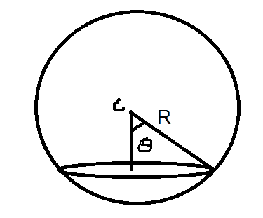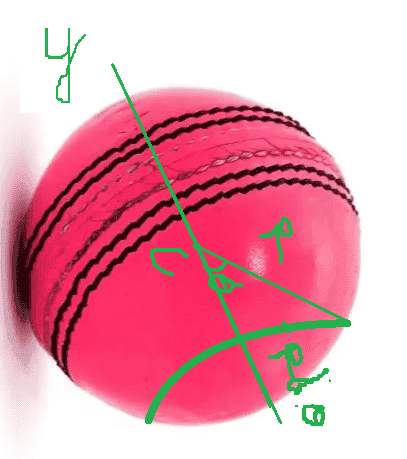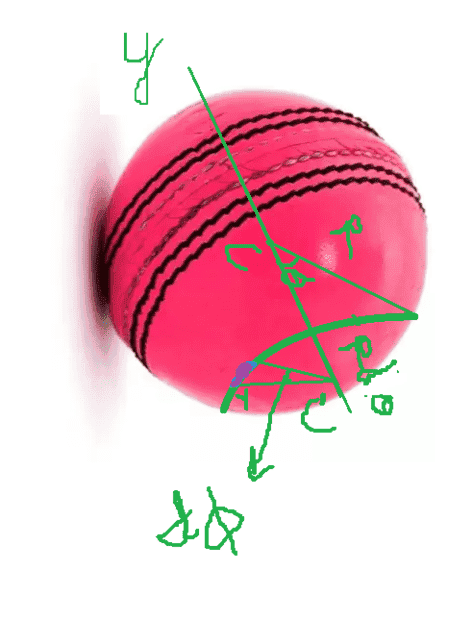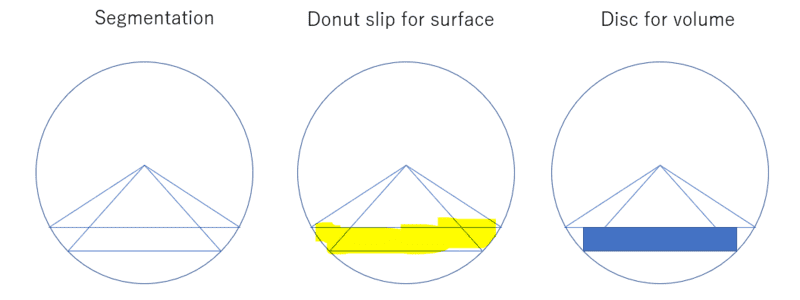# Deriving volume of a sphere

• Pushoam
In summary, the area of a disc of thickness ##R d\theta## is given by:$$A = \int^{\pi}_{0} \pi R^2 sin^2 \theta R d\theta$$

#### Pushoam

Homework Statement
Derive the volume of a sphere.
Relevant Equations
$$V = \int^{2\pi}_{0}\int^{\pi}_{0}\int^{R}_{0} r^{2}sin \theta drd\phi d\theta$$I consider a disc of thickness ## R d\theta ## as shown in the figure.
Then, $$dV = \pi R^2 sin^2 \theta R d\theta$$ ( Area of the disc * its thickness)
Hence, $$V = \int^{\pi}_{0} \pi R^2 sin^2 \theta R d\theta$$
$$V = \frac 1 {2} {\pi}^2 R^3$$ ....(1)

While $$V = \int^{2\pi}_{0}\int^{\pi}_{0}\int^{R}_{0} r^{2}sin \theta drd\phi d\theta = \frac 4 {3} {\pi} R^3$$ ...(2)

Could you please tell me what mistakes I have committed in (1)?

Last edited:
Thickness or Height of disk is ##R \sin \theta d\theta## which is multiplied by area to give volume.

•Pushoam
To elaborate a bit on what @anuttarasammyak said, the volume of the disk is ##dV = (\pi x^2)\, dy## where ##x = R \sin\theta## and ##y = -R\cos\theta##.

•PushoamIf I take thickness along y-axis then, it is ## dy = R sin \theta d \theta ## as $$y = -R cos \theta$$ $$\frac {dy}{d \theta} = R sin \theta$$ $$dy = R sin \theta d \theta$$.
But, if I take thickness along the radial direction, then it is ## R d \theta##. For example, if I want to derive its surface area, then I consider the green ring with radius ## R sin \theta ## and thickness ## d \theta##. I consider the region bounded by this ring as a disc. Hence, the radius and thickness of the ring should be ## R sin\theta ## and ## R d\theta## respectively, shouldn't it?

Pushoam said:
But, if I take thickness along the radial direction, then it is Rdθ.
Thickness or height should be perpendicular to area. ##Rd\theta## is perimeter length along the circle, not height.

anuttarasammyak said:
Thickness or height should be perpendicular to area. ##Rd\theta## is perimeter length along the circle, not height.In the figure, C' is the centre of the ring. The perimeter length segment is the violet colour part, which is ## r d \phi##, where ## r = R sin \theta ##. Hence, the perimeter is ## 2 \pi R sin\theta##.

## R d\theta ## is the thickness of the ring.

Area of this ring = ## 2 \pi R sin\theta R d\theta ##

Hence, area of sphere = ## \int_0^{\pi }2 \pi R sin\theta R d\theta = 4 \pi R^2 ##.

Which is your concern, area or volume ? Slip or disk ? Please find attached my sketch for them.•Pushoam
Pushoam said:
View attachment 320782In the figure, C' is the centre of the ring. The perimeter length segment is the violet colour part, which is ## r d \phi##, where ## r = R sin \theta ##. Hence, the perimeter is ## 2 \pi R sin\theta##.

## R d\theta ## is the thickness of the ring.
Not the thickness.

This measures distance along the surface of the sphere for this ring segment.
Area of this ring = ## 2 \pi R sin\theta R d\theta ##
But this surface area is along the surface of the sphere.
Hence, area of sphere = ## \int_0^{\pi }2 \pi R sin\theta R d\theta = 4 \pi R^2 ##.
Right. This gives you the surface area of the sphere which demonstrates that you have not been using the thickness (a vertical measurement) of each disk, but rather a distance along the sphere's surface.

•Pushoam
anuttarasammyak said:
Which is your concern, area or volume ? Slip or disk ? Please find attached my sketch for them.View attachment 320784
My concern is: while calculating surface area, ## R d\theta ## is taken as the thickness of the ring, but while calculating volume ## R d\theta ## is not taken as the thickness of the disc bounded by the ring. Why is it so?
It seems thickness of the ring and thickness of the disc are different quantities and I am mistaking in taking them same.

SammyS said:
Not the thickness.

This measures distance along the surface of the sphere for this ring segment.

But this surface area is along the surface of the sphere.

Right. This gives you the surface area of the sphere which demonstrates that you have not been using the thickness (a vertical measurement) of each disk, but rather a distance along the sphere's surface.
Thanks for the clarification. I got it.

•SammyS
Pushoam said:
My concern is: while calculating surface area, Rdθ is taken as the thickness of the ring, but while calculating volume Rdθ is not taken as the thickness of the disc bounded by the ring. Why is it so?
It seems thickness of the ring and thickness of the disc are different quantities and I am mistaking in taking them same.
I prefer to say ##Rd\theta## is width not thickness of ring in order to avoid confusion. Word thickness would be applied to 3D objects. Here ring is 2D slip we use to calculate area. English mother tongue colleagues would help us.

•Pushoam
anuttarasammyak said:
I prefer to say ##Rd\theta## is width not thickness of ring in order to avoid confusion. Word thickness would be applied to 3D objects. Here ring is 2D slip we use to calculate area. English mother tongue colleagues would help us.
Thanks for clarification.

Pushoam said:
My concern is: while calculating surface area, ## R d\theta ## is taken as the thickness of the ring, but while calculating volume ## R d\theta ## is not taken as the thickness of the disc bounded by the ring. Why is it so?
It seems thickness of the ring and thickness of the disc are different quantities and I am mistaking in taking them same.
Neither are rings.
The disc is a disc, and as with any solid to get the volume you multiply an area by its extent normal to the area.
The surface area element is a frustrated cone. It has a perimetric length, and as with any 2D manifold to get the area you multiply the length by the manifold’s extent normal to the length.

•Pushoam
Thanks

anuttarasammyak said:
Here ring is 2D slip we use to calculate area. English mother tongue colleagues would help us.
I can't tell what you mean by "slip" from your drawings. Possibly "strip" would be a better choice of words, meaning the portion of the surface of the sphere delimited by two parallel slices of the sphere, that are relatively close together.

•anuttarasammyak